Function Repository Resource:

# RandomDate

Sample random date lists

Contributed by: Michael Sollami
 ResourceFunction["RandomDate"][] gives a random date in the current year. ResourceFunction["RandomDate"][n] gives n random dates in the current year. ResourceFunction["RandomDate"][{low, high}] generates a random date between low and high. ResourceFunction["RandomDate"][{low,high},n] generates n random dates in the given range.

## Details and Options

ResourceFunction["RandomDate"] date ranges should be given as lists.
ResourceFunction["RandomDate"] returns lists of DateObject expressions.

## Examples

### Basic Examples (5)

Generate some random dates:

 In:=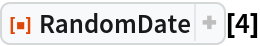Out=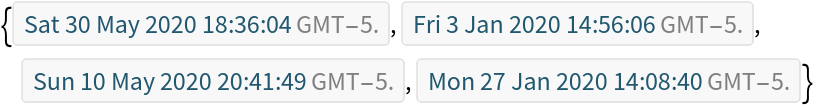Generate a tensor of random dates:

 In:=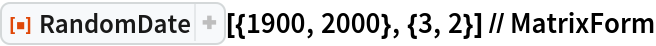Out=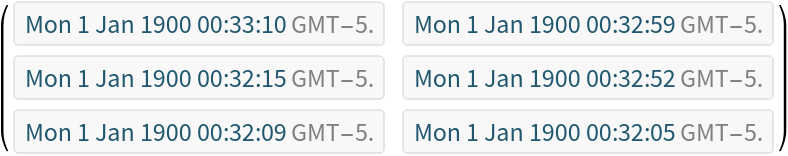Generate random dates between days:

 In:=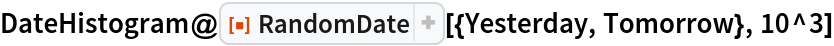Out=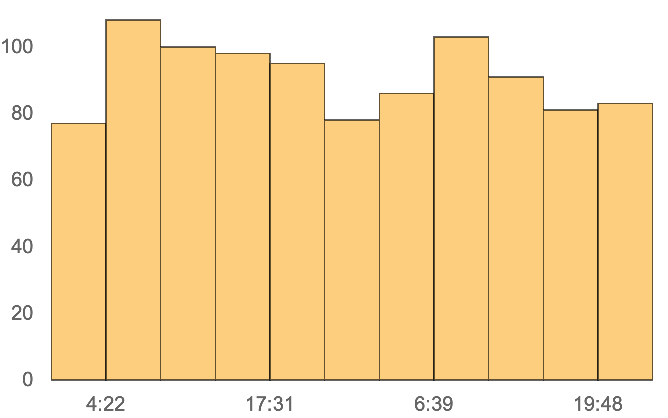Plot lists of random dates:

 In:=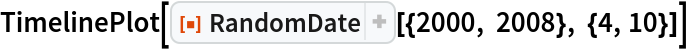Out=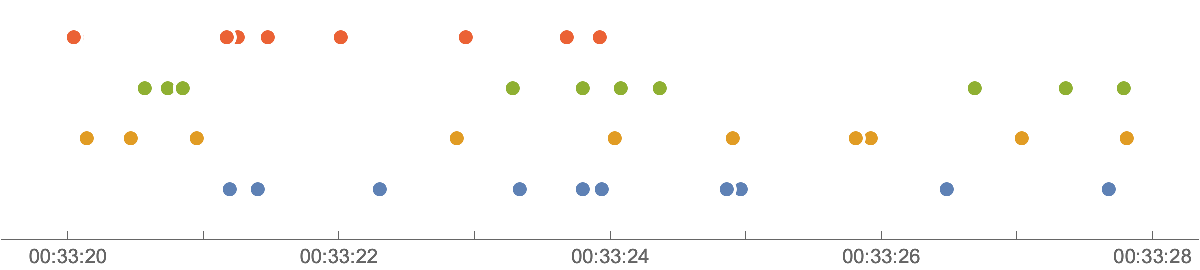Make a timeline plot of random dates in the first quarter of the year:

 In:=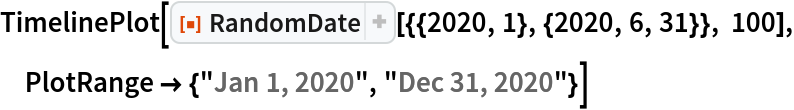Out=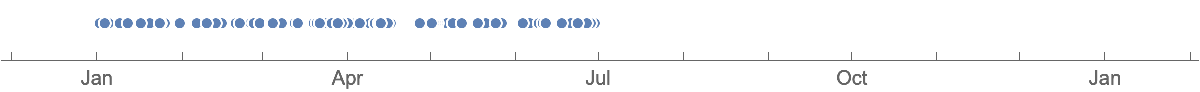Michael Sollami

## Version History

• 1.0.0 – 07 April 2020